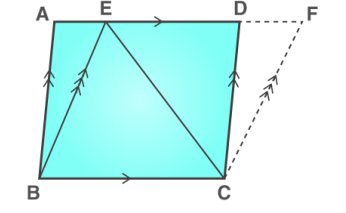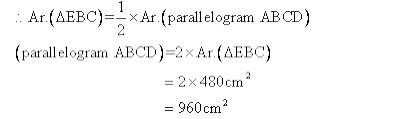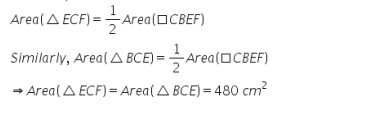Selina solutionsOur top 5% students will be awarded a special scholarship to Lido.
Set your child up for success with Lido, book a class today!

. ABCD and BCFE are parallelograms. If area of triangle EBC=480cm2 , AB=30cm and BC=40cm; Calculate;

(i) Area of parallelogram ABCD;

(ii) Area of parallelogram BCFE;

(iii) Length of altitude from A on CD;

(iv) Area of triangle ECF.Looking to do well in your science exam ? Learn from an expert tutor. Book a free class!

(i) Since △ EBC and parallelogram ABCD are on the same base BC and between the same parallels i.e. BC//AD.(ii)

Parallelograms on the same base and between the same parallels are equal in area

Area of BCFE = Area of ABCD= 960 cm2

(iii)

Area of triangle ACD=480 = (1/2) x 30 x Altitude

Altitude=32 cm

(iv)

The area of a triangle is half that of a parallelogram on the same base and between the same parallels.

Therefore,Our top 5% students will be awarded a special scholarship to Lido.Lido

Courses

Teachers

Book a Demo with us

Syllabus

Science
English
Coding

Terms & Policies

NCERT Question Bank

Maths
Science

Selina Question Bank

Maths
Physics
Biology

Allied Question Bank

Chemistry